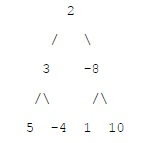# Minimum sum path between two leaves of a binary trees in C++

## Problem statement

Given a binary tree in which each node element contains a number. The task is to find the minimum possible sum from one leaf node to another.

## ExampleIn above tree minimum sub path is -6 as follows: (-4) + 3 + 2 + (-8) + 1

## Algorithm

The idea is to maintain two values in recursive calls −

• Minimum root to leaf path sum for the subtree rooted under current node
• The minimum path sum between leaves
• For every visited node X, we have to find the minimum root to leaf sum in left and right sub trees of X. Then add the two values with X’s data, and compare the sum with the current minimum path sum

## Example

Live Demo

#include <bits/stdc++.h>
using namespace std;
typedef struct node {
int data;
struct node *left;
struct node *right;
} node;
node *newNode(int data) {
node *n = new node;
n->data = data;
n->left = NULL;
n->right = NULL;
return n;
}
int getMinPath(node *root, int &result) {
if (root == NULL) {
return 0;
}
if (root->left == NULL && root->right == NULL) {
return root->data;
}
int leftSum = getMinPath(root->left, result);
int rightSum = getMinPath(root->right, result);
if (root->left && root->right) {
result = min(result, root->data + leftSum + rightSum);
return min(root->data + leftSum, root->data + rightSum);
}
if (root->left == NULL) {
return root->data + rightSum;
} else {
return root->data + leftSum;
}
}
int getMinPath(node *root) {
int result = INT_MAX;
getMinPath(root, result);
return result;
}
node *createTree() {
node *root = newNode(2);
root->left = newNode(3);
root->right = newNode(-8);
root->left->left = newNode(5);
root->left->right = newNode(-4);
root->right->left = newNode(1);
root->right->right = newNode(10);
return root;
}
int main() {
node *root = createTree();
cout << "Minimum sum path = " << getMinPath(root) << endl;
return 0;
}

When you compile and execute above program. It generates following output −

## Output

Minimum sum path = -6